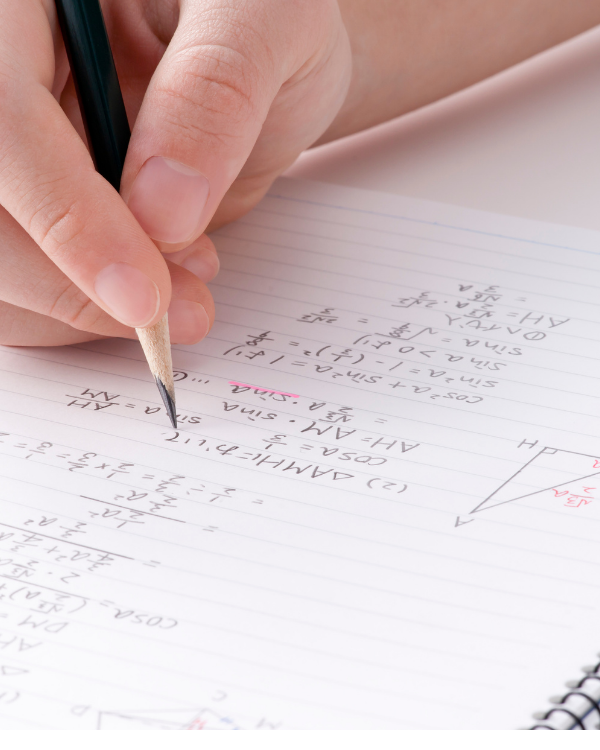# A LEVEL FURTHER MATHEMATICSOverviewExamsFurther Progression

## Course Overview

This Pearson Edexcel Level 3 Advanced GCE in Further Mathematics builds on the skills, knowledge and understanding set out in the whole GCSE subject content for mathematics and the subject content for the Pearson Edexcel Level 3 Advanced Subsidiary and Advanced GCE Mathematics qualifications.

### Students will learn to:

• understand mathematics and mathematical processes in ways that promote confidence, foster enjoyment and provide a strong foundation for progress to further study
• extend their range of mathematical skills and techniques
• understand coherence and progression in mathematics and how different areas of mathematics are connected
• apply mathematics in other fields of study and be aware of the relevance ofmathematics to the world of work and to situations in society in general
• use their mathematical knowledge to make logical and reasoned decisions in solving problems both within pure mathematics and in a variety of contexts, and communicate the mathematical rationale for these decisions clearly
• reason logically and recognise incorrect reasoning
• generalise mathematically
• construct mathematical proofs
• use their mathematical skills and techniques to solve challenging problems which require them to decide on the solution strategy
• recognise when mathematics can be used to analyse and solve a problem in context
• represent situations mathematically and understand the relationship between problems in context and mathematical models that may be applied to solve them
• draw diagrams and sketch graphs to help explore mathematical situations and interpret solutions
• make deductions and inferences and draw conclusions by using mathematical reasoning
• interpret solutions and communicate their interpretation effectively in the context of the problem
• read and comprehend mathematical arguments, including justifications ofmethods and formulae, and communicate their understanding## Exam Overview

The Pearson Edexcel Level 3 Advanced GCE in Further Mathematics consists of four externally-examined papers. Students must take Paper 1 and Paper 2, the two mandatory Core Pure papers, and two optional papers. Students are permitted to take more than the two optional papers if they want to extend their course of study.

##### Duration

Paper 1: 1 hour and 30 minutes Paper 2: 1 hour and 30 minutes Optional Paper 1: 1 hour and 30 minutes Optional Paper 2: 1 hour and 30 minutes

##### Format

External assessment offered two times a year for each of the four papers.

##### Components

Paper 1: 75 marks Paper 2: 75 marks Optional Paper 1: 75 marks Optional Paper 2: 75 marks

Created By CGA
Created By CGA